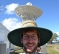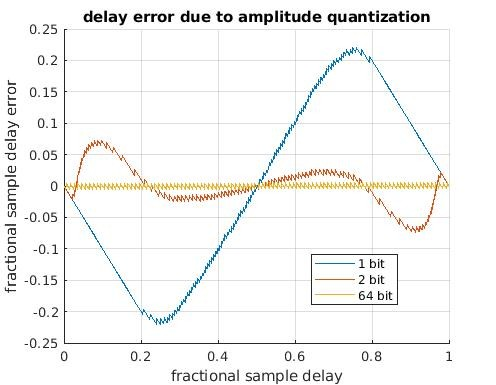## delay error due to quantizationStarted by 3 years ago2 replieslatest reply 3 years ago140 views

Hi guys,

I have two random signals (created by matlab randn), where the second signal represents only a delayed version (by a fraction of an integer) of the first one:

x2[n] = x1[n-fd]

n ... discrete time index 0,1,2,3,4....N

fd ... fractional delay (0,1)

When I correlate the two signal and look for the fractional sample delay I obtain exactly the same value.

As soon I apply a amplitude quantization (1 and 2 bit) of the signals x1 and x2, the fractional sample delay I obtain by correlation is different. (see the plot below)

Plot description:

When I compare the input fraction delay with correlated delay (difference on the y-axis) for various fraction delays between 0 and 1 (x-axis) I see this patterns for 1 and 2 bit quantization. The 64 bit representation of the two signals (no quantization) shows no difference. So there is only a difference once I apply amplitude quantization.Is this a known error when applying a quantization of a noise signal? If yes, would it be possible to point me to some literature or explain this behaviour?

Best regards,

Jakob

[ - ]Your x1 is generated from randn

your x2 is generated from FD of x1 (how?, do you apply FD filter).

if my samples are 0 and 1 only(1 bit) I don't see how I can get a value in between 0 & 1

[ - ]